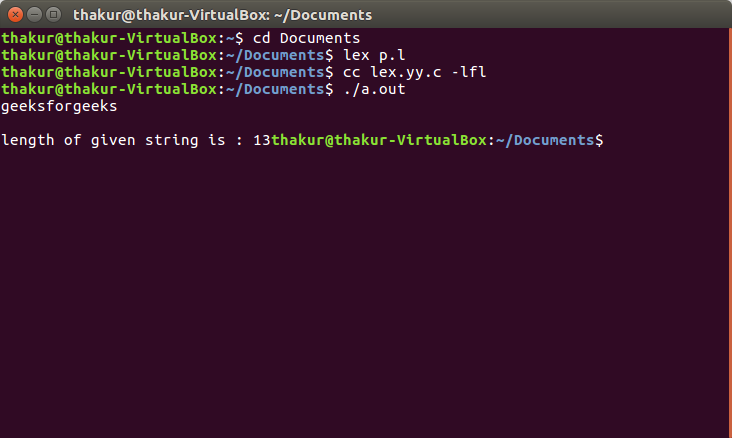Open in App
Not now

# Lex program to find the Length of a String

• Difficulty Level : Basic
• Last Updated : 30 Apr, 2019

Problem: Write a Lex program to find the Length of a String

Explanation:
FLEX (Fast Lexical Analyzer Generator) is a computer program that generates lexical analyzers and was written by Mike Lesk and Eric Schmidt. Lex reads an input stream specifying the lexical analyzer and outputs source code implementing the lexer in the C programming language.

Examples:

```Input: geeksforgeeks
Output: length of given string is : 13

Input: geeks
Output: length of given string is : 5 ```

Implementation:

 `/*lex program to find the length of a string*/` ` `  `%{ ` `  ``#include  ` `  ``int`  `length; ` `%} ` ` `  `/* Rules Section*/` `%% ` `  ``[a-z A-Z 0-9]+ {length=yyleng; } ` `%% ` ` `  `int` `main() ` ` ``{ ` `  ``yylex(); ` `  ``printf``(``"length of given string is : %d"``, length); ` `  ``return` `0; ` ` ``} `

Output:My Personal Notes arrow_drop_up
Related Articles